# Mix¶

## Allanon¶

Blends the upper layer as half-transparent with the lower. (It add the two layers together and then halves the value).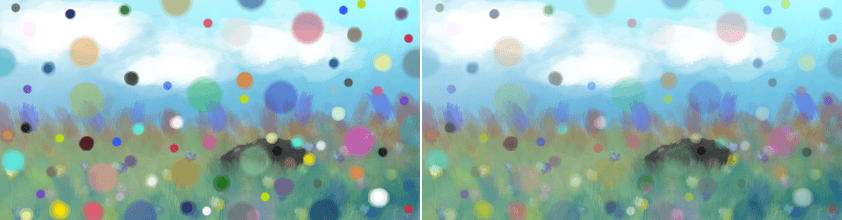Left: Normal. Right: Allanon.

## Interpolation¶

Subtract 0.5f by 1/4 of cosine of base layer subtracted by 1/4 of cosine of blend layer assuming 0-1 range. The result is similar to Allanon mode, but with more contrast and functional difference to 50% opacity.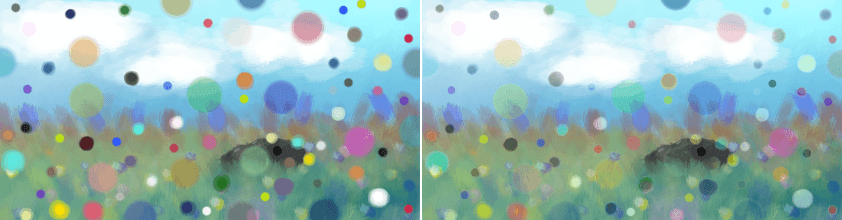Left: Normal. Right: Interpolation.

## Interpolation - 2X¶

Applies Interpolation blend mode to base and blend layers, then duplicate to repeat interpolation blending.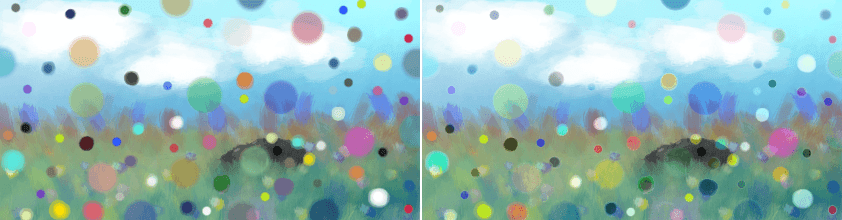Left: Normal. Right: Interpolation - 2X.

## Alpha Darken¶

As far as I can tell this seems to premultiply the alpha, as is common in some file-formats.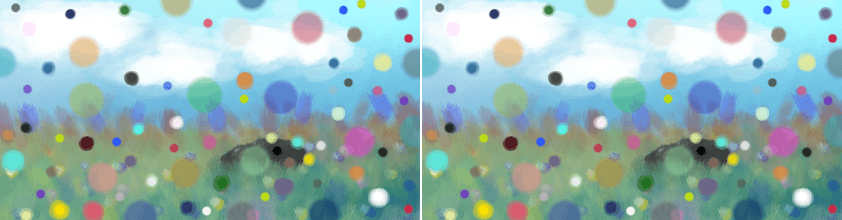Left: Normal. Right: Alpha Darken.

## Behind¶

Does the opposite of normal, and tries to have the upper layer rendered below the lower layer.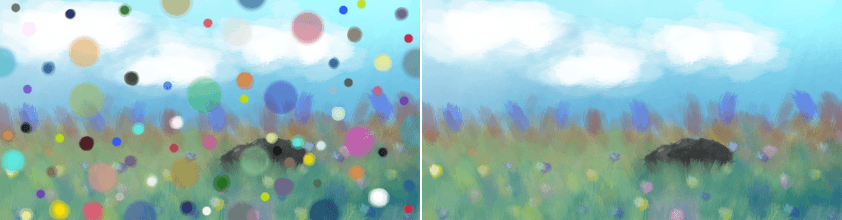Left: Normal. Right: Behind.

## Erase¶

This subtracts the opaque pixels of the upper layer from the lower layer, effectively erasing.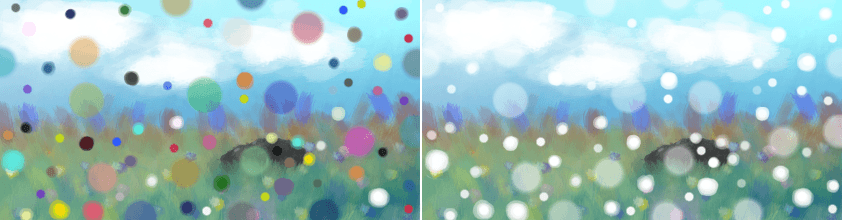Left: Normal. Right: Erase.

## Geometric Mean¶

This blending mode multiplies the top layer with the bottom, and then outputs the square root of that.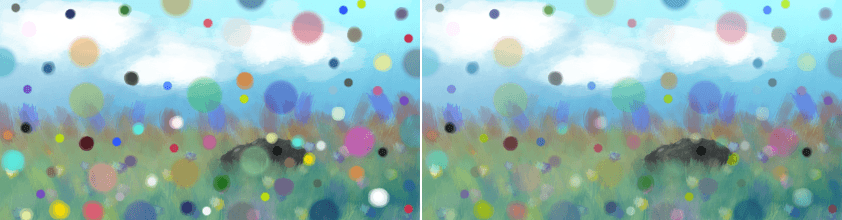Left: Normal. Right: Geometric Mean.

## Grain Extract¶

Similar to subtract, the colors of the upper layer are subtracted from the colors of the lower layer, and then 50% gray is added.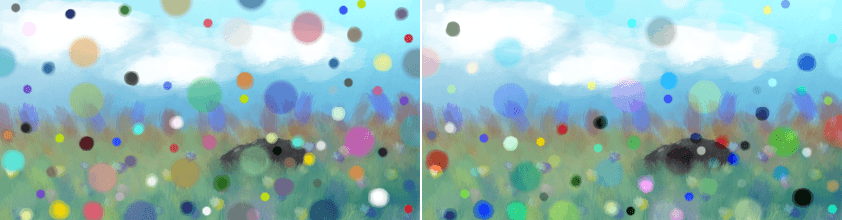Left: Normal. Right: Grain Extract.

## Grain Merge¶

Similar to addition, the colors of the upper layer are added to the colors, and then 50% gray is subtracted.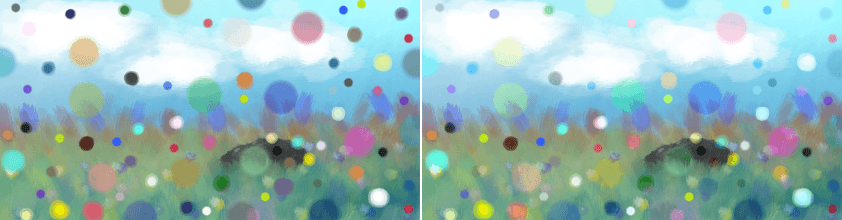Left: Normal. Right: Grain Merge.

## Greater¶

A blending mode which checks whether the painted color is painted with a higher opacity than the existing colors. If so, it paints over them, if not, it doesn't paint at all.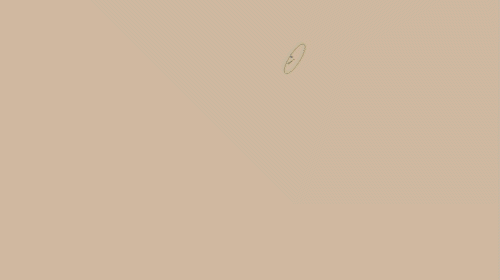## Hard Mix¶

Similar to Overlay.

Mixes both Color Dodge and Burn blending modes. If the color of the upper layer is darker than 50%, the blending mode will be Burn, if not the blending mode will be Color Dodge.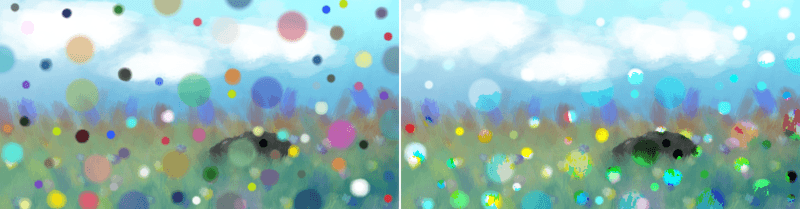Left: Normal. Right: Hard Mix.

## Hard Mix (Photoshop)¶

This is the hard mix blending mode as it is implemented in photoshop.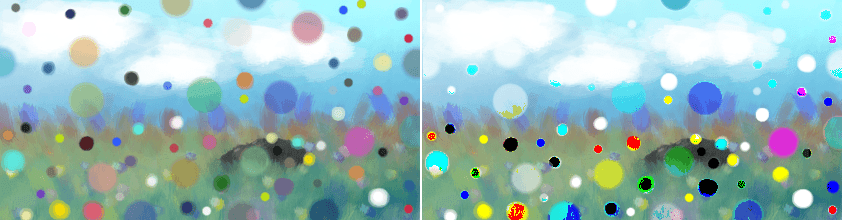Left: Dots are mixed in with the normal blending mode, on the Right: Dots are mixed in with hardmix.

This add the two values, and then checks if the value is above the maximum. If so it will output the maximum, otherwise the minimum.

## Hard Overlay¶

バージョン 4.0 で追加.

Similar to Hard light but hard light use Screen when the value is above 50%. Divide gives better results than Screen, especially on floating point images.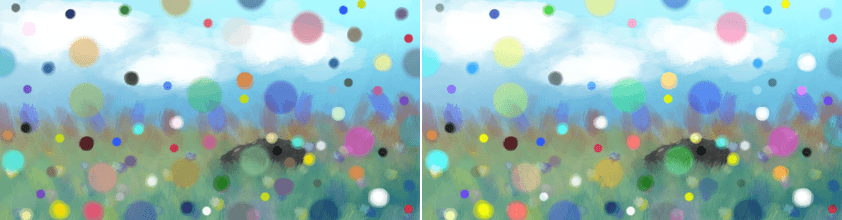Left: Normal. Right: Hard Overlay.

## Normal¶

As you may have guessed this is the default Blending mode for all layers.

In this mode, the computer checks on the upper layer how transparent a pixel is, which color it is, and then mixes the color of the upper layer with the lower layer proportional to the transparency.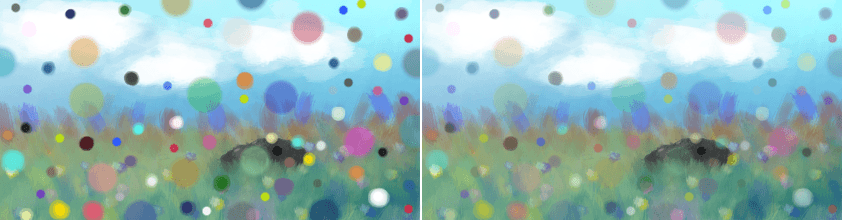Left: Normal 100% Opacity. Right: Normal 50% Opacity.

## Overlay¶

A combination of the Multiply and Screen blending modes, switching between both at a middle-lightness.

Overlay checks if the color on the upperlayer has a lightness above 0.5. If so, the pixel is blended like in Screen mode, if not the pixel is blended like in Multiply mode.

This is useful for deepening shadows and highlights.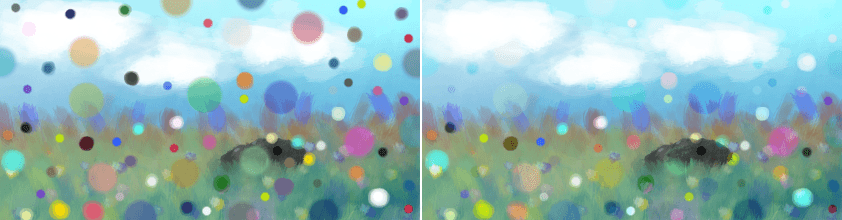Left: Normal. Right: Overlay.

## Parallel¶

This one first takes the percentage in two decimal behind the comma for both layers. It then adds the two values. Divides 2 by the sum.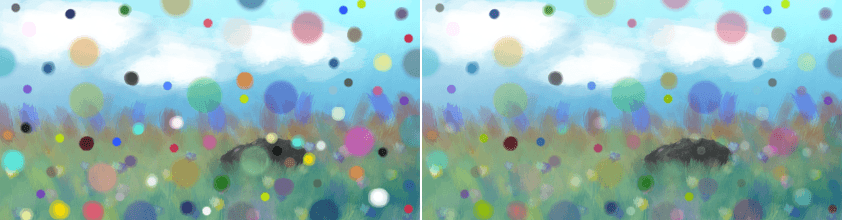Left: Normal. Right: Parallel.

## Penumbra A¶

Creates a linear penumbra falloff. This means most tones will be in the midtone ranges.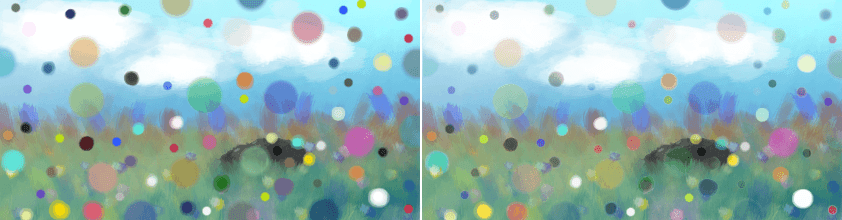Left: Normal. Right: Penumbra A.

## Penumbra B¶

Penumbra A with source and destination layer swapped.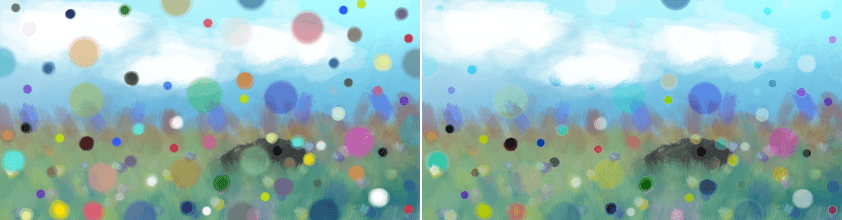Left: Normal. Right: Penumbra B.

## Penumbra C¶

Creates a penumbra-like falloff using arc-tangent formula. This means most tones will be in the midtone ranges.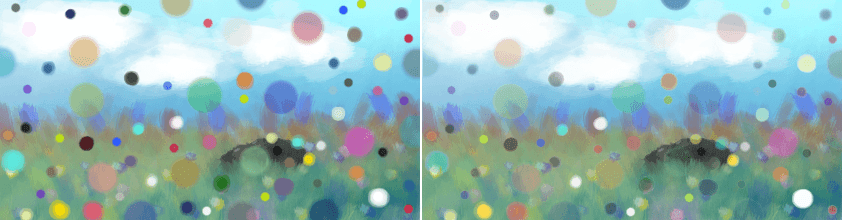Left: Normal. Right: Penumbra C.

## Penumbra D¶

Penumbra C with source and destination layer swapped.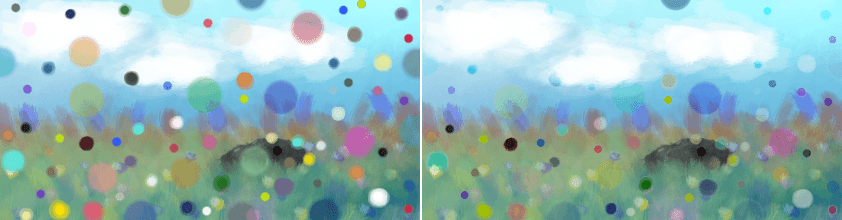Left: Normal. Right: Penumbra D.